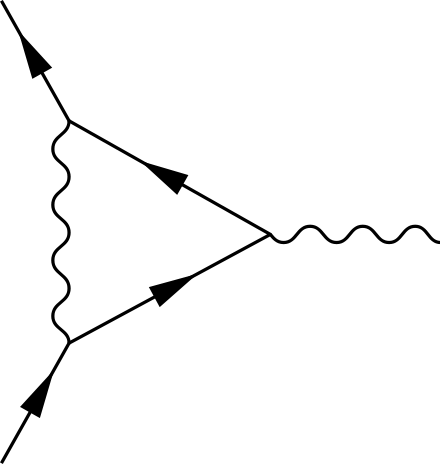# Are You Magnetic?

It’s no secret that the face of particle physics lies in the collaboration of scientists all around the world – and for the first time a group of 170 physicists have come to a consensus on one of the most puzzling predictions of the Standard Model muon. The anomalous magnetic moment of the muon concerns the particle’s rotation, or precession, in the presence of a magnetic field. Recall that elementary particles, like the electron and muon, possess intrinsic angular momentum, called spin, and hence indeed behave like a little dipole “bar magnet” – consequently affected by an external magnetic field.

The “classical” version of such an effect comes straight from the Dirac equation, a quantum mechanical framework for relativistic spin-1/2 particles like the electron and muon. It is expressed in terms of the g-factor, where$g=2$ in the Dirac theory. However, more accurate predictions, to compare to with experiment, require more extended calculations in the framework of quantum field theory, with “loops” of virtual particles forming the quantum mechanical corrections. In such a case we of course find deviation from the classical value in what becomes the anomalous magnetic moment with$a = \frac{g-2}{2}$

For the electron, the prediction coming from Quantum Electrodynamics (QED) is so accurate, it actually agrees with the experimental result up to 10 significant figures (side note: in fact, this is not the only thing that agrees very well with experiment from QED, see precision tests of QED).Figure 1: a “one-loop” contribution to the magnetic dipole moment in the theory of Quantum Electrodynamics (QED)

The muon, however, isn’t so simple and actually gets rather messy. In the Standard Model it comes with three parts, QED, electroweak and hadronic contributions$a^{SM}_{\mu} = a^{QED}_{\mu}+a^{EW}_{\mu}+a^{hadron}_{\mu}$

Up until now, the accuracy of these calculations have been the subject of a number of collaborations around the world. The largest source (in fact, almost all) of the uncertainty actually comes from the smaller contributions to the magnetic moment, the hadronic part. It is so difficult to estimate that it actually requires input from experimental sources and lattice QCD methods. This review constitutes the most comprehensive report of both the data-driven and lattice methods for hadronic contributions to the muon’s magnetic moment.

Their final result,$a^{SM}_{\mu} = 116591810(43) \times 10^{-11}$ remains 3.7 standard deviations below the current experimental value, measured at Fermilab in Brookhaven National Laboratory. However the most exciting part about all this is the fact that Fermilab is on the brink of releasing a new measurement, with the uncertainties reduced by almost a factor of four compared to the last. And if they don’t agree then? We could be that much closer to confirmation of some new physics in one of the most interesting of places!